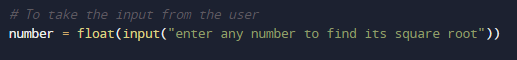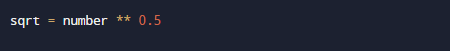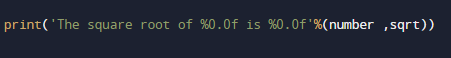# Python Program to Find the Square Root

In this tutorial you will learn about the Python Program to Find the Square Root and its application with practical example.

In this tutorial, we will learn to create a Python Program to Find the Square Root using Python Programming.

## Prerequisites

Before starting with this tutorial we assume that you are best aware of the following Python programming topics:

• Python Operator.
• Basic Input and Output
• Basic Python  programming.
• Python if-else statements.
• Python Loop.

## What is Square root?

So the Squares root of a number is found when number is multiplying the number itself twice 6 * 6= 36. Where a square root of a number on getting multiplied by itself gives the original √36 = 6. A square root of a numbera’ is a number b such that =a.

## Python Program to Find the Square Root

In our program, we will create a Python Program that Find the Square Root of a given number.First we would declared and initialized the required variables. Here in this program we are going find Square root of a given number first of all we take a value from user and pass this value to in-built function to find Square root of a number.

## Outputin our program, we have first declared and initialized a set variables required in the program.

• number= it will hold value given by user.
• sqrt= it will hold square root of a number

First of all in our program we will take all required variable and value, and then take value from user  at run time and the value given by user will be convert to int from string  (as in python the value we take from input from user are always string so we need to convert it into int) and will be stored into variable called ‘number’.After taking these values from user we will calculate the square root a given number.Find the square root by applying  the “**” exponent operator.our program only works for Positive real numbers.And at last  after calculating Square root finally we will print the value of variable ‘sqrt’ to get our desired result.i.e square root of a number.In this tutorial we have learn about the Python Program to Find the Square Root and its application with practical example. I hope you will like this tutorial.